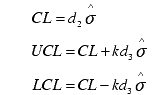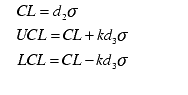# Range chart calculations

Range chart control limits are calculated as follows:

 Control Limits calculated from data Control Limits calculated from standard deviation (s ) are given)NOTE: If subgroup size is variable, m is replaced with mi for ith subgroup limits. When subgroup size is variable, the control limit values will not be the same; therefore, the chart may display `city skyline' type control lines (when subgroup size is from 2 - 25).

## Data Plot Items

• Subgroup Range

• Lower Control Limit (LCL) (optional)

• Upper Control Limit (UCL) (optional)

• Warning lines (optional)

## Descriptive Statistics

• Lower Control Limit (LCL)

• Upper Control Limit (UCL)

• Center Line (CL)